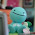## Friday, January 14, 2011

### Final Percent Post

Percent means out of a hundred. It's another name for hundredths. They can be represented as a fraction or a decimal.
This chapter, we did percents. We have learned a lot from, showing percents on a hundred grid(s), converting them into decimals and fractions, combining percents and a percent of a number.

We first learned about representing percents. You can have percents from less than 0 to more than 100 on a grid . The percents involve fractions as well.
An example of a number on a hundred grid, containing more than 100 is:An example of a number on a hundred grid, containing less than 100 is:The next thing we learned about fractions were converting them into fractions and decimals.
Example: convert the following into a decimal and percents .
a) 3/40 = 0.075
the numerator divided by the denominator and you get the decimal .
b) 0.075 x 100 = 7.5

4.3 was finding a percent of a number. I like to use the ratio grid to find the percent .
example: whatever you do one side, you do to the other side.The last thing we learned about percents were combining them . I thought this was really easy.

#### 1 comment:

1.I like how you used a picture to explain what you were talking about. You should add some colour so it can stick out a bit more. Your pictures were very understandable and easy to read great job Allison!